Numerical analysis of the drag reduction for turbulent pulsating pipe flows based on large eddy simulations
NING Tao, GU ChunweiKey Laboratory for Thermal Science and Power Engineering of Ministry of Education, Department of Thermal Engineering, Tsinghua University, Beijing 100084, China
Abstract: Large eddy simulations were conducted for turbulent pulsating flows using the commercial solver ANSYS-CFX. The drag reduction and the total energy consumption for pulsating flows were analyzed. The simulations included current dominated and wave dominated pulsating flows. The boundary layer characteristics of the current flow were affected by the superposition of the wave flow. The best drag reduction in the pulsating flows gave a 25% drag reduction when the non-dimensional pulsating amplitude was 5.5. The analysis indicates that the drag reduction is optimized when the pulsating flow is wave dominated and the wave boundary layer is laminar. Pulsating flows with simple sinusoidal pulsating patterns consume much more energy than steady flows.
Key words: pulsating pipe flows     turbulence     large eddy simulation     drag reduction     energy consumption

 ${{\mathop{\rm \mathit{Re}}\nolimits} _{\rm{C}}} = VD/{U_{\rm{m}}}, {{\mathop{\rm \mathit{Re}}\nolimits} _{\rm{W}}} = U_{\rm{m}}^2/\left( {\omega v} \right), \alpha = {U_{\rm{m}}}/V.$ (1)

1 研究对象

 算例 ReC ReW α 稳态流 6 087 0 0 脉动流1 6 077 103 0.25 脉动流2 6 116 2 470 1.3 脉动流3 6 834 62 629 5.5 脉动流4 6 019 280 321 13

2 数值模拟方法 2.1 数值方法

Scotti等采用大涡数值模拟对槽道脉动湍流的研究中，通过与直接数值模拟(direct numerical simulation, DNS)以及实验的对比，确认了Dynamic Smagorinsky亚格子应力模式能够很好地预测脉动湍流。故本文采用ANSYS软件包中的CFX作为求解器，选用Dynamic Smagorinsky亚格子应力模式对管流进行大涡数值模拟。其中:扩散项离散采用中心型格式，时间项离散采用二阶Euler后差格式。

2.2 边界条件

2.3 网格划分

 $\left\{ {\begin{array}{*{20}{c}} {{\rm{流向}}\;\;\;\;\;\;\;\;\;\;\;\;\;\;\Delta {z^ + } = \Delta z{u_\tau }/v, }\\ {{\rm{径向}}\;\;\;\;\;\;\;\;\;\;\;\;\;\;\;\Delta {r^ + } = \Delta r{u_\tau }/v, }\\ {{\rm{周向}}\;\;\;\;\;\;\;{{\left( {r\Delta \theta } \right)}^ + } = \left( {r\Delta \theta } \right){u_\tau }/v.} \end{array}} \right.$ (2)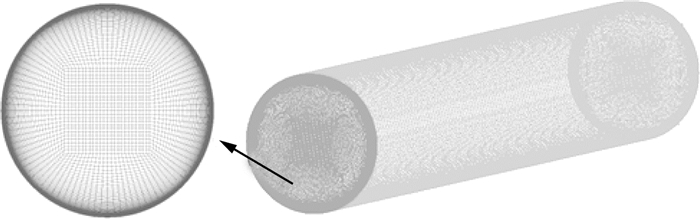图 1 计算网格示意

 网格总数 Δz+ Δr+ (rΔθ)+ Δt+ 920 623 20.0 0.02~6.6 6.6~12.6 7×10－5

3 计算结果及分析 3.1 计算验证

 ${U^ + } = V/{u_\tau }, T = \sqrt {u'u'} /{u_\tau }.$ (3)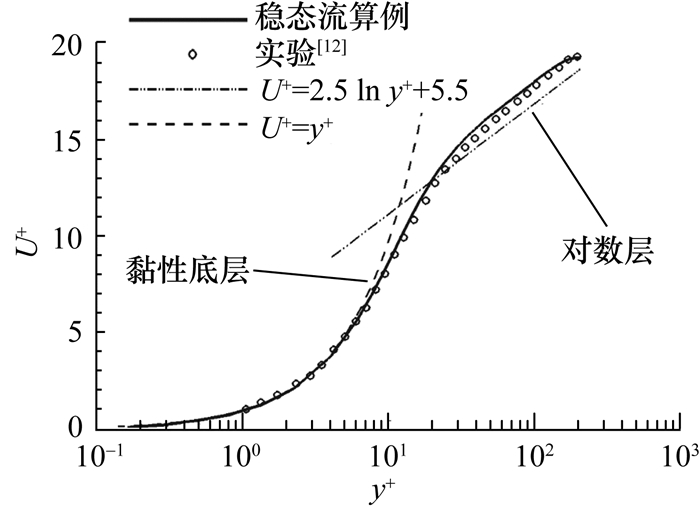图 2 量纲为1的速度剖面图 3 沿径向的湍动度对比

3.2 减阻分析

 ${D_{\rm{R}}} = \left( {{C_{{\rm{fBlasius}}}}-{C_{\rm{f}}}} \right)/{C_{{\rm{fBlasius}}}}.$ (4)

 算例 ReC ReW α DR/% 脉动流1 6 077 103 0.25 5 脉动流2 6 116 2 470 1.3 10 脉动流3 6 834 62 929 5.5 25 脉动流4 6 091 280 321 13 18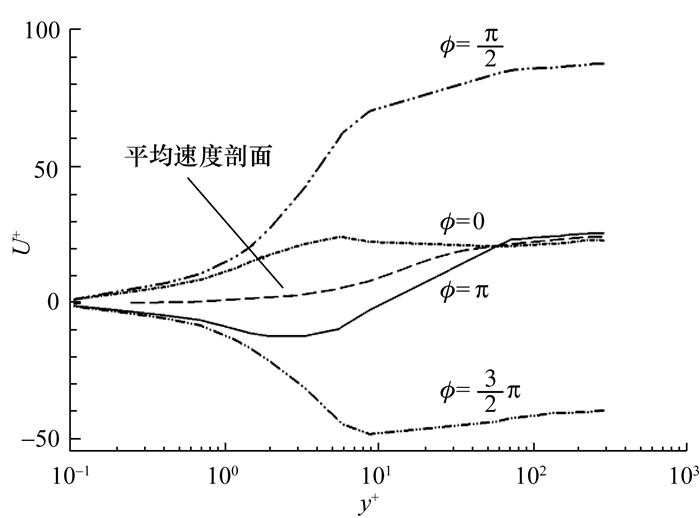图 4 不同相位的相平均速度剖面(α=5.5)图 5 稳态流和脉动流的Reynolds应力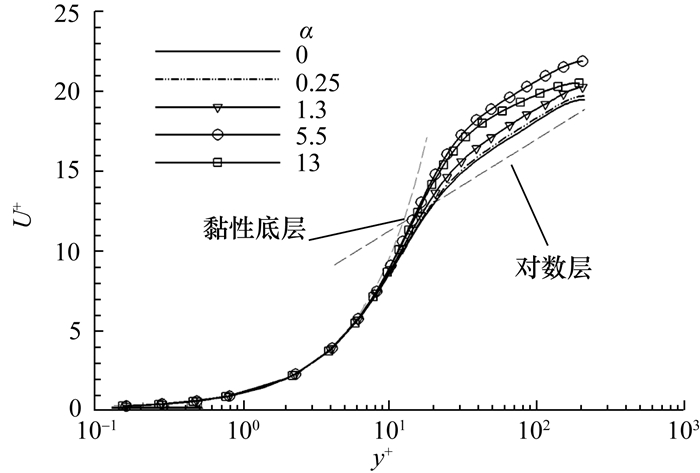图 6 不同算例的速度剖面比较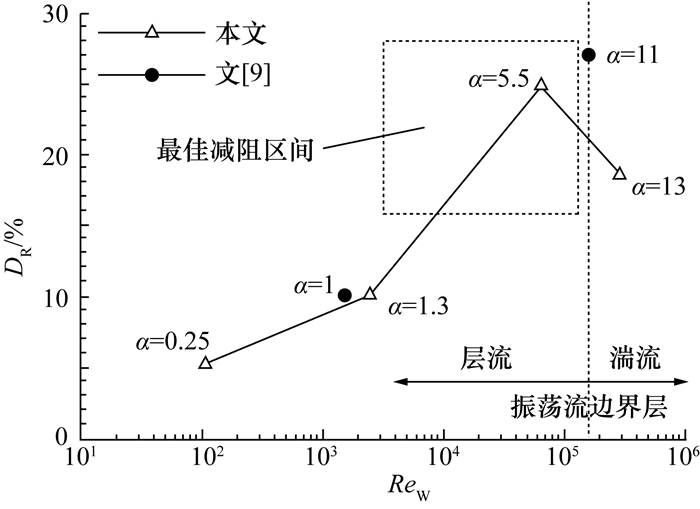图 7 大涡模拟得到的减阻率比较

3.3 总能耗评估

 $\left\{ {\begin{array}{*{20}{c}} {{E_{\rm{P}}} = \frac{{\int_0^{2{\rm{\pi }}} {u\left( \phi \right)\Delta p\left( {1 + \beta \sin \phi } \right)R{\rm{d}}\phi } }}{{2{\rm{\pi }}\rho L{V^3}}}, }\\ {{E_{\rm{S}}} = \frac{{\int_0^{2{\rm{\pi }}} {V\Delta pR{\rm{d}}\phi } }}{{2{\rm{\pi }}\rho L{V^3}}} = \frac{{\Delta pR{\rm{d}}\phi }}{{\rho L{V^2}}}.\;\;\;\;\;\;} \end{array}} \right.$ (5)

 算例 α EP ES EP/ES 脉动流1 0.25 0.009 8 0.008 1.13 脉动流2 1.3 0.035 6 0.008 4.15 脉动流3 5.5 0.472 7 0.006 68.90 脉动流4 13.0 3.087 4 0.008 9 349.0

4 结论

1) 本文计算的脉动流算例包括稳态流主控和振荡流主控两种流态，在脉动幅值α=5.5时获得了显著的减阻效果，减阻率DR为25%。

2) 脉动流主要通过叠加合适的振荡流来改变稳态流边界层的特性从而达到减阻目的。当振荡流分量的边界层为层流且振荡流分量主控脉动流流态时，稳态湍流分量有被振荡流分量层流化的趋势。因此，当脉动流的流态由振荡流主控且振荡流分量的边界层为层流时，减阻效果较好。

3) 脉动流的总能耗高于相应的稳态流，采用非常规的脉动形式有减小总能耗的潜力。要将脉动流作为实际工程应用中的一种减阻手段还需要进一步研究。

  Lodahl C R, Sumer B M, Fredsoe J. Turbulent combined oscillatory flow and current in a pipe[J]. Journal of Fluid Mechanics, 1998, 373: 313–348. DOI:10.1017/S0022112098002559  MAO Zhuoxiong, Hanratty T J. Studies of the wall shear stress in a turbulent pulsating pipe flow[J]. Journal of Fluid Mechanics, 1986, 170: 545–564. DOI:10.1017/S0022112086001015  Akhavan R, Kamm R D, Shapiro A H. An investigation of transition to turbulence in bounded oscillatory stokes flows, Part 1. Experiments[J]. Journal of Fluid Mechanics, 1991, 225: 395–422. DOI:10.1017/S0022112091002100  Tu S W, Ramaprian B R. Fully developed periodic turbulent pipe flow: Part 1. Main experimental results and comparison with predictions[J]. Journal of Fluid Mechanics, 1983, 137: 31–58. DOI:10.1017/S0022112083002281  Ramaprian B R, Tu S W. Fully developed periodic turbulent pipe flow: Part 2. The detailed structure of the flow[J]. Journal of Fluid Mechanics, 1983, 137: 59–81. DOI:10.1017/S0022112083002293  He S, Jackson J D. An experimental study of pulsating turbulent flow in a pipe[J]. European Journal of Mechanics-B: Fluids, 2009, 28(2): 309–320. DOI:10.1016/j.euromechflu.2008.05.004  Tuzi R, Blondeaux P. Intermittent turbulence in a pulsating pipe flow[J]. Journal of Fluid Mechanics, 2008, 599: 51–79.  Scotti A, Piomelli U. Numerical simulation of pulsating turbulent channel flow[J]. Physics of Fluids, 2001, 13(5): 1367–1384. DOI:10.1063/1.1359766  Manna M, Vacca A. Resistance reduction in pulsating turbulent pipe flows[J]. Journal of Engineering for Gas Turbines and Power, 2005, 127: 410–417. DOI:10.1115/1.1789511  Manna M, Vacca A. Spectral dynamic of pulsating turbulent pipe flow[J]. Computers & Fluids, 2008, 37(7): 825–835.  Tardu S F, Binder G, Blackwelder R F. Turbulent channel flow with large-amplitude velocity oscillations[J]. Journal of Fluid Mechanics, 1994, 267: 109–151. DOI:10.1017/S0022112094001138  Eggels J G M, Unger F, Weiss M H, et al. Fully developed turbulent pipe flow: A comparison between direct numerical simulation and experiment[J]. Journal of Fluid Mechanics, 1994, 268: 175–210. DOI:10.1017/S002211209400131X  Souma A, Iwamoto K, Murata A. Experimental analysis of pressure-gradient profile upon drag-reduction effect in pulsating turbulent pipe flow[J]. Transactions of the Japan Society of Mechanical Engineers Series B, 2012, 78(787): 521–530. DOI:10.1299/kikaib.78.521